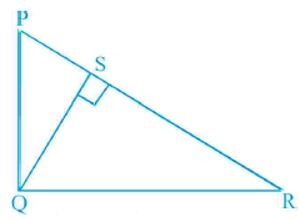#### In Fig., PQR is a right triangle right angled at Q and . If PQ = 6 cm and PS = 4 cm, find QS, RS and QR.Given: PQR is a triangle

PQ = 6 cm, PS = 4 cm

In

(common angles and each angle is 90°)

(each equal to )

( by AA similarity criterion)

By cross multiply we get

In use Pythagoras theorem.

Put in equation (1)

In use Pythagoras theorem